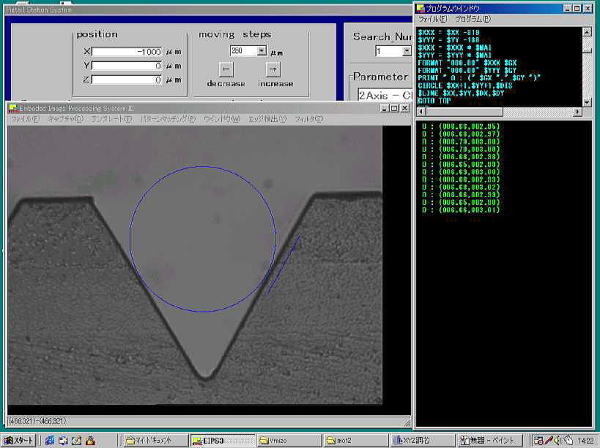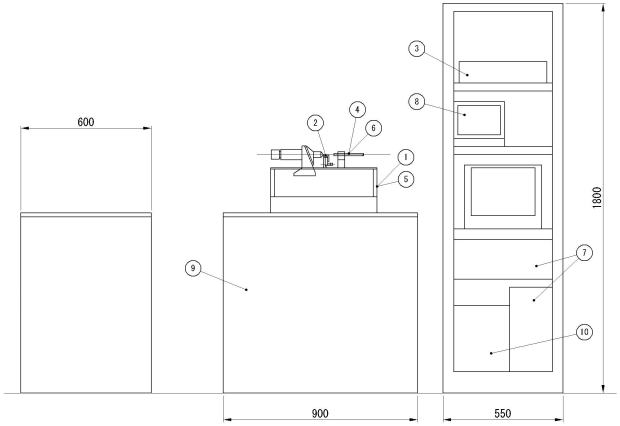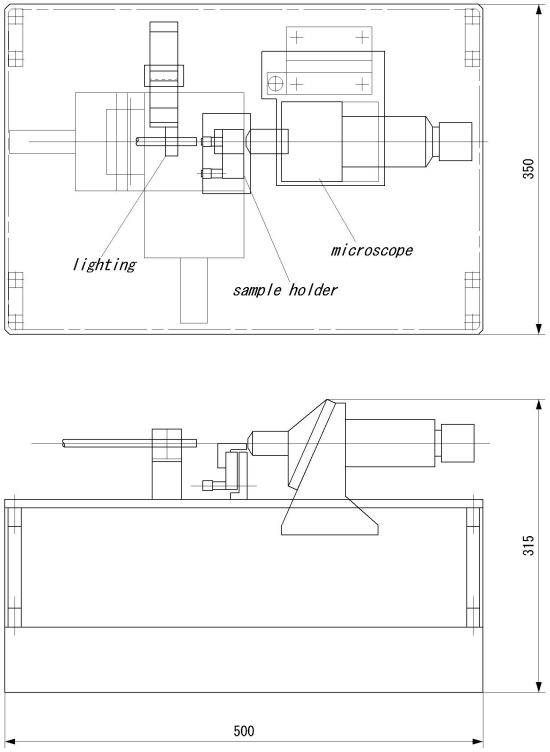# Core measurement machine specification

###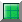Outline

This machine is developed for core pitch measurement of fiber array.
Ultra high precise measurement is achieved by using CCD camera, laser scale and image processing.
With an option, this machine can measure the direction and the divergent angle of the beam of collimator array.

###Constitution

1) xy stage

 xy stage is consist of highly precise ball screw and highly precise slide rail, and the max speed of table is 1mm/sec. x axis stroke +/- 10 mm resolution 0.05 micron y axis stroke +/- 10 mm resolution 0.05 micron max speed 1mm/sec

2) sample holder

 mechanical cramp

3) image processing department

 CCD camera 400,000 picture element, B/W tact time 12 seconds for 8 fiber array. recognition speed 0.3 - 0.5 sec function pattern matching calculation of center of gravity position

 *note: Tact time is according to averaging sample number. With an option, 1,500,000 picture element CCD camera, accuracy grows up much more precise.

4) high-resolution scope

 objective lens 20X with revolver CCD camera 400,000 picture element, B/W holder xy manual operation

5) scale

 laser scale minimum dial 0.01 micron made by Sony stroke 20 mm

6) lighting

 lighting for core white lamp lighting for V groove optical fiber lighting

7) motor controller

 controllable axis 2 axis maximum application frequency 16 kHz

8) monitor for CCD camera

 size 9 inch, B/W

9) vibration absorber

 air pressure 4 kg/cm2 adjustment auto absorber performance 8 Hz or more

10) option

 auto manipulator for collimator 1,500,000 picture element CCD camera image processing system for false wearing prevention

11) guarantee

 inconvenience by our responsibility: one year In the case of foreign countries, extra trip charge is needed.

## DATA SAMPLE 1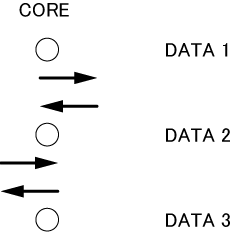### DATA 3

center =(000.55,-000.09)
center =(000.57,-000.14)
center =(000.52,-000.07)
center =(000.54,-000.12)
center =(000.57,-000.14)
center =(000.54,-000.07)
center =(000.56,-000.10)
center =(000.56,-000.15)
center =(000.53,-000.05)
center =(000.54,-000.09)
center =(000.57,-000.15)
center =(000.54,-000.06)
center =(000.56,-000.08)
center =(000.57,-000.14)
center =(000.53,-000.05)
center =(000.56,-000.10)
center =(000.57,-000.15)
center =(000.53,-000.06)
center =(000.55,-000.09)
center =(000.57,-000.15)
center =(000.53,-000.05)
center =(000.54,-000.09)
center =(000.56,-000.14)
center =(000.54,-000.05)
center =(000.55,-000.11)
center =(000.58,-000.16)
center =(000.53,-000.05)
center =(000.54,-000.11)
center =(000.55,-000.15)
center =(000.53,-000.06)
center =(000.55,-000.10)
center =(000.54,-000.16)
center =(000.54,-000.07)
center =(000.55,-000.10)
center =(000.57,-000.18)
center =(000.54,-000.06)
center =(000.55,-000.09)
center =(000.55,-000.18)
center =(000.53,-000.06)
center =(000.55,-000.09)
center =(000.56,-000.16)
center =(000.54,-000.06)
center =(000.56,-000.10)
center =(000.57,-000.15)
center =(000.54,-000.05)
center =(000.56,-000.11)
center =(000.57,-000.15)
center =(000.53,-000.06)

## DATA SAMPLE 2 (V GROOVE)